Next: Installation of GRTOOL Up: GRTOOL A Link-to-Grid Previous: Introduction

In this section we briefly describe the basic terms and concepts used in the link-to-grid interface implemented in GRTOOL.

A link is considered a straight line connection between two network nodes. For each link a, the coordinates of the I-node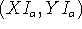and of the J-node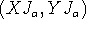are known from the network definition. A width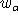is associated with each link. It is specified in the same units as the network coordinates and serves to define the geometric link representation, which is a centered link bar of widthextending between I- and J-node. For each link a set of data values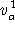,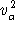,, ..., represents the data that is to be transformed to the grid level.

A grid is a subdivision of the network plane by equally spaced horizontal and vertical parallel lines. The distance between two neighboring parallel lines is called the grid distance. It is defined in the same units as the network coordinates. The relative position of the parallels is such that a horizontal and a vertical line always pass through the origin of the coordinate system, i.e. point (0,0). The resulting square shaped subdivisions are called grid cells. They are identified by their lower left corner coordinates.

Each link value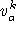is split proportionally to the area overlap with the corresponding grid cells. The resulting partial values are then accumulated for each grid cell. The fact that the link is represented as a two-dimensional bar, instead of a one-dimensional line segment, avoids any ambiguities that might otherwise arise when a link coincides with a cell boundary or passes through a cell corner. This is illustrated in the following figure, which shows how the data for a given link (shown in solid black) is distributed to the neighboring grid cells: Since, depending on the network size and the chosen grid distance, the link-to-grid conversion can be quite a complex task, an efficient implementation is very important. The following is an outline of the method implemented in grlink:

1. The link data file is read and, for each link, the coordinates of the corresponding link bar are computed. Using a recursive approach, the bar is intersected with all intervening grid cells and the intersection areas are computed. For each grid cell that intersects with the link bar a record is written to a temporary file containing the grid cell coordinates and the corresponding shares of the data values.
2. The temporary file containing the link specific grid records is sorted according to the grid cell coordinates, resulting in another temporary file in which all data concerning one grid cell is now contiguous.
3. The sorted temporary file is read sequentially. For each grid cell, the corresponding data values are accumulated and the totals are written into the grid data file.Next: Installation of GRTOOL Up: GRTOOL A Link-to-Grid Previous: Introduction

Heinz Spiess, EMME/2 Support Center
Sun Mar 3 22:21:06 MET 1996